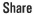01. 02. 03. 04. 05. 06. 07. 08. 09. 10. 11. 12. 13. 14. 15. 16. 17. 18. 19. 20. 21. 22. 23. 24. 25. 26. 27. 28. 29. 30. 31. 32. 33. 34. 35. 36. 37. 38. 39. 40. 41. 42. 43. 44. 45. 46. 47. 48. 49. 50. 51. 52. 53. 54. 55. 56. 57. 58. 59. 60. 61. 62. 63. 64. 65. 66. 67. 68. 69. 70. 71. 72. 73. 74. 75. 76. 77. 78. 79. 80. 81. 82. 83. 84. 85. 86. 87. 88. 89. 90. 91. 92. 93. 94. 95. 96. 97. 98. 99. 100. 101. 102. 103. 104. 105. 106. 107. 108. 109. 110. 111. 112. 113. 114. 115. 116. 117. 118. 119. 120. 121. 122. 123. 124. 125. 126. 127. 128. 129. 130. 131. 132. 133. 134. 135. 136. 137. 138. 139. 140. 141. 142. 143. 144. 145. 146. 147. 148. 149. 150. 151. 152. 153. 154. 155. 156. 157. 158. 159. 160. 161. 162. 163. 164. 165. 166. 167. 168. 169. 170. 171. 172. 173. 174. 175. 176. 177. 178. 179. 180. 181. 182. 183. 184. 185. 186. 187. 188. 189. 190. 191. 192. 193. 194. 195. 196. 197. 198. 199. 200. 201. 202. 203. 204. 205. 206. 207. 208. 209. 210. 211. 212. 213. 214. 215. 216. 217. 218. 219. 220. 221. 222. 223. 224. 225. 226. 227. 228. 229. 230. 231. 232. 233. 234. 235. 236. 237. 238. 239. 240. 241. 242. 243. 244. 245. 246. 247. 248. 249. 250. 251. 252. 253. 254. 255. 256. 257. 258. 259. 260. 261. 262. 263. 264. 265. 266. 267. 268. 269. 270. 271. 272. 273. 274. 275. 276. 277. 278. 279. 280. 281. 282. 283. 284. 285. 286. 287. 288. 289. 290. 291. 292. 293. 294. 295. 296. 297. 298. 299. 300. 301. 302. 303. 304. 305. 306. 307. 308. 309. 310. 311. 312. 313. 314. 315. 316. 317. 318. 319. 320. 321. 322. 323. 324. 325. 326. 327. 328. 329. 330. 331. 332. 333. 334. 335. 336. 337. 338. 339. 340. 341. 342. 343. 344. 345. 346. 347. 348. 349. 350. 351. 352. 353. 354. 355. 356. 357. 358. 359. 360. 361. 362. 363. 364. 365. 366. 367. 368. 369. 370. 371. 372. 373. 374. 375. 376. 377. 378. 379. 380. 381. 382. 383. 384. 385. 386. 387. 388. 389. 390. 391. 392. 393. 394. 395. 396. 397. 398. 399. 400. 401. 402. 403. 404. 405. 406. 407. 408. 409. 410. 411. 412. 413. 414. 415. 416. 417. 418. 419. 420. 421. 422. 423. 424. 425. 426. 427. 428. 429. 430. 431. 432. 433. 434. 435. 436. 437. 438. 439. 440. 441. 442. 443. 444. 445. 446. 447. 448. 449. 450. 451. 452. 453. 454. 455. 456. 457. 458. 459. 460. 461. 462. 463. 464. 465. 466. 467. 468. 469. 470. 471. 472. 473. 474. 475. 476. 477. 478. 479. 480. 481. 482. 483. 484. 485. 486. 487. 488. 489. 490. 491. 492. 493. 494. 495. 496. 497. 498. 499. 500. 501. 502. 503. 504. 505. 506. 507. 508. 509. 510. 511. 512. 513. 514. 515. 516. 517. 518. 519. 520. 521. 522. 523. 524. 525. 526. 527. 528. 529. 530. 531. 532. 533. 534. 535. 536. 537. 538. 539. 540. 541. 542. 543. 544. 545. 546. 547. 548. 549. 550. 551. 552. 553. 554. 555. 556. 557. 558. 559. 560. 561. 562. 563. 564. 565. 566. 567. 568. 569. 570. 571. 572. 573. 574. 575. 576. 577. 578. 579. 580. 581. 582. 583. 584. 585. 586. 587. 588. 589. 590. 591. 592. 593. 594. 595. 596. 597. 598. 599. 600. 601. 602. 603. 604. 605. 606. 607. 608. 609. 610. 611. 612. 613. 614. 615. 616. 617. 618. 619. 620. 621. 622. 623. 624. 625. 626. 627. 628. 629. 630. 631. 632. 633. 634. 635. 636. 637. 638. 639. 640. 641. 642. 643. 644. 645. 646. 647. 648. 649. 650. 651. 652. 653. 654. 655. 656. 657. 658. 659. 660. 661. 662. 663. 664. 665. 666. 667. 668. 669. 670. 671. 672. 673. 674. 675. 676. 677. 678. 679. 680. 681. 682. 683. 684. 685. 686. 687. 688. 689. 690. 691. 692. 693. 694. 695. 696. 697. 698. 699. 700. 701. 702. 703. 704. 705. 706. 707. 708. 709. 710. 711. 712. 713. 714. Image 86 of 714To start/stop a slide show click on Start/Stop above. To go to the next image on your own just click on the image

Back to Main Front Page